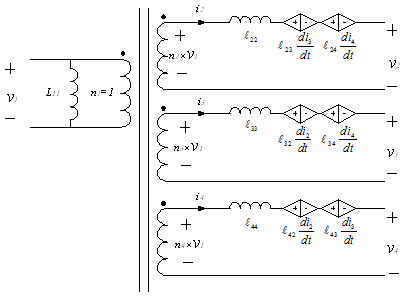LIBRARY

# A Simplified Model for Tightly Coupled Transformer with Multiple WindingsFig. 1. N-port model for 4-winding transformer.
This paper presents a new model for multiple-winding transformer to tackle the effect of leakage phenomenon on converter operation in a designer friendly form. Based on the basic mutual inductance equations, the N-port model is derived. Further, a linear model is derived based on tightly coupling assumption, i.e. coupling coefficients between winding pairs are more than 0.9.

A schematic diagram for 4-winding transformer in this N-port cross-regulation model is shown in Fig. 1. In this model, the input side has a parallel inductor, which equates to the self-inductance of the primary winding. The secondary side output voltages are the sums of the primary-side voltage times effective turn ratio, the voltage drop on leakage inductance, and all sensed voltages of cross-regulation inductance from other secondary windings. If the winding resistance is not negligible, corresponding winding resistors should be placed in serial with each winding into this model.